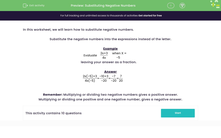# Substitute Negative Numbers into Algebraic Expressions

In this worksheet, students will substitute negative numbers into algebraic expressions.Key stage:  KS 3

Curriculum topic:   Algebra

Curriculum subtopic:   Understand Expressions, Equations, Inequalities, Terms and Factors

Difficulty level:#### Worksheet Overview

In this activity, we will learn how to substitute negative numbers.

Substitute the negative numbers into the expressions instead of the letter.

Example

Evaluate
 2x + 3 4x
when X = -5

 2(-5) + 3 4(-5)
=
 -10 + 3 -20
=
 -7 -20
=
 7 20

Multiplying or dividing two negative numbers gives a positive answer.

Multiplying or dividing one positive and one negative number, gives a negative answer.

Let's have a go at some questions.

### What is EdPlace?

We're your National Curriculum aligned online education content provider helping each child succeed in English, maths and science from year 1 to GCSE. With an EdPlace account you’ll be able to track and measure progress, helping each child achieve their best. We build confidence and attainment by personalising each child’s learning at a level that suits them.

Get started